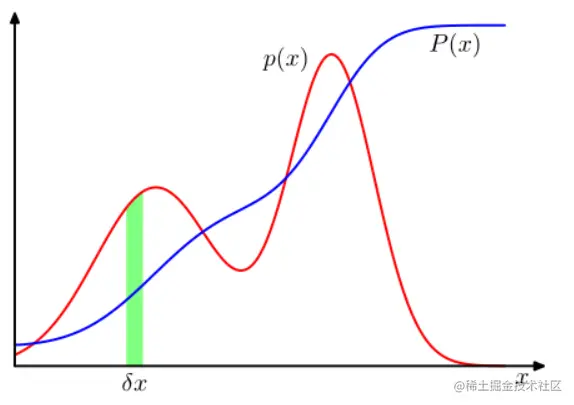# 18-19 (1.2.1 probability density)

2022-01-27 05:03:12 CNpupil

### 1.2.1 Probability density

In addition to considering the probability defined on the discrete-time set , We also want to consider the probability of continuous variables . We will confine ourselves to relatively informal discussions . If the real valued variable $x$​ Fall in the range $(x,x+\delta x)$​ The probability within is determined by $p(x)\delta x$ Express $\delta x\rightarrow 0$, that $p(x)$ be called $x$​ Probability density on .​​ Pictured 1.12 Shown . $x$ In the interval $(a,b)$ The probability within is given by ：

$p(x\in(a,b))=\int_a^bp(x)dx\tag{1.24}$

Because of the probability yes Nonnegative , And because $x$ The value of must be somewhere on the real axis , So probability density $p(x)$ These two conditions must be met

$p(x)\geq0\tag{1.25}$
$\int_{-\infty}^\infty p(x)dx=1\tag{1.26}$

Under the nonlinear variation of variables , Due to the Jacobian factor , Probability density transformation is different from simple function . for example , If we consider variables $x=g(y)$ The change of , So the function $f(x)$ Turn into $f(y)=f(g(y))$. Now consider the probability density $p_x(x)$, It corresponds to the new variable $y$ The density of the $p_y(y)$, Where enough means $p_x(x)$ and $p_y(y)$ It's a fact of different densities . For the smaller $\delta x$ Value , be located $(x,x+\delta x)$ Observations in the range will be converted to $(y,y+\delta y)$ Range , among $p_x(x)\delta x \simeq p_y(y)\delta y$​, therefore

$P(z)=\int_{-\infty}^zp(x)dx\tag{1.28}$

It meets the $P'(x)=p(x)$​​, Pictured 1.12 Shown .chart 1.12 discrete The probability of the variable Can be diffused into continuous variables $x$ Probability density on $p(x)$, And make it in the interval $(x,\delta x)$ Of $x$ The probability of is determined by $\delta x$ Of $p(x)\delta x$ give $\delta x\rightarrow0$. The probability density can be expressed as a cumulative distribution function $P(x)$ The derivative of .

If we have several continuous variables $x_1,...,x_D$, With the vector $x$ Express , Then we can define the joint probability density $p(x)=p(x_1,...,x_D)$ bring x Fall into inclusion point $x$ Infinitesimal volume of $\delta x$ The probability in is determined by $p(x)\delta x$ give . This multivariate probability density must satisfy

$p(x)\geq0\tag{1.29}$
$\int p(x)dx=1\tag{1.30}$

The integral is for the whole $x$​ Integral of space . We can also consider the combination of joint probability distribution on discrete variables and continuous variables .

Be careful , If $x$ It's a discrete variable , that $p(x)$ Sometimes referred to as the probabilistic mass function , Because it can be seen as a group focused on $x$​ At the allowable value “ Probability quality ”.

Probability sum product rule and Bayesian theorem are also applicable to the case of probability density , Or a combination of discrete and continuous variables . for example , If $x$ and $y$ Are two real variables , Then the form of sum rule and product rule is

$p(x)=\int p(x,y)dy\tag{1.31}$
$p(x,y)=p(y|x)p(x)\tag{1.32}$

The formal proof of the sum product rule of continuous variables requires a branch of mathematics called measurement theory , Not within the scope of this book . However , By dividing each real variable into width intervals , Its effectiveness can be seen informally $\Delta$ Consider the discrete probability distributions on these intervals . Walking limit $\Delta\rightarrow0$​ Then convert the sum to Integrate and give the desired result .